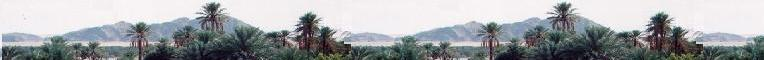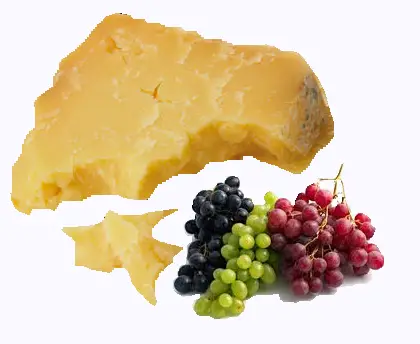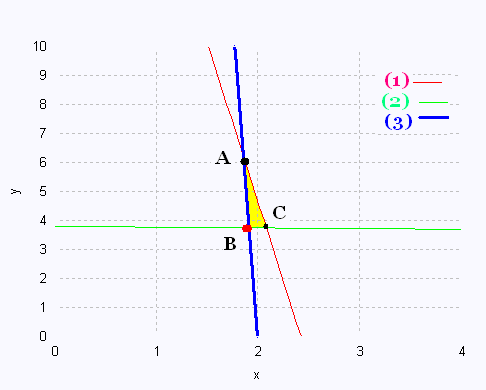Mathematics 2: Linear optimization Examples

Balanced dietJim weights 72.5 kg. To balance his diet, he needs daily, en average, 80 g of protein, 300 g of carbohydrates (carb), and 88 g of lipids (fat).

He will take just cheese and seedless raisins along a day, by using two recipes:

1. Nutrient 1:
100 g Cheddar Cheese nutrition facts:
Protein: 33 g
Carb: 2 g
Fat: 44 g
Price: 4.00$and 2. Nutrient 2: 100 g Seedless raisins nutrition facts: Protein: 3 g Carb: 79 g Fat: 1 g Price: 1.00$

How many grammes of each nutrient Jim will buy to maintain a balanced diet and pay less.

x is the number of nutrient 1, and
y is the number of nutrient 2.

(1) 33 x + 3 y ≥ 80
(2) 2 x + 79 y ≥ 300
(3) 44 x + y ≥ 88

(1) y = - 11 x + 80/3
(2) y = - 2/79 x + 300/79
(3) y = - 44 x + 88

Z = 4 x + 1 y minThe 3 vertices are:

1. A(1.86,6.22)
- 11 x + 80/3 = - 44 x + 88
x = 1.86
y = 6.22

2. B(1.92, 3.70)
- 2/79 x + 300/79 = - 44 x + 88
x = 1.92
y = 3.70

3. C(2.10, 3.67)

- 11 x + 80/3 = - 2/79 x + 300/79
x = 2.10
y = 3.67

 Vertex Z ($) (1.86, 6.22) 13.74 (1.92, 3.70) 11.38 (2.10, 3.67) 12.07 To obtain the minimum price to pay, which is 11.38$, Jim will take 1.92 x 100 g = 192 g of Cheddar cheese , and 3.70 x 100 g = 370 g of raisins to keep a balanced diet for one day.Web ScientificSentence

 chimie labs | scientific sentence | java | php | green cat | contact |